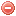# Description

The $$I_{87}$$ was developed by Carrega (1988, 1991) in order to predict fire danger in Southern France. It is based on the principle that fire occurrence and fire spread are conditioned by the combined effects of wind, air humidity, temperature and soil water reserves (i.e. surface and deep reserves). The $$I_{87}$$ requires thus air temperature and relative humidity, wind speed, and potential evapotranspiration (according to Thornthwaite 1948) as input variables. It also includes a phenological coefficient in order to take seasonal differences in vegation condition into account (Carrega 1988).

# Formula

The $$I_{87}$$ is calculated as follows Carrega 1988):

$I_{87}=\dfrac{1}{\sqrt{r}\cdot{r_s}}\cdot{max[10, T]}\cdot\dfrac{U}{H}\cdot{PC}$

where $$r$$ is the deep water reserve [mm] corresponding to the Orieux water reserve $$r$$ (cf. Orieux 1974), $$r_s$$ the surface water reserve [mm], $$T$$ air temperature [°C], $$U$$ mean wind speed [m/s], $$H$$ relative air humidity [%], and $$PC$$ a phenological coefficient.

$$PC$$ equals 200 in winter and in summer when $$r$$ is smaller than 50 [mm] or when grass is yellowed, 100 in spring and in autumn when grass is green.

Carrega (1988) set the maximum surface water reserve $$r_s$$ to 10 [mm], and assumed that $$r_s$$ decreases exponentially as a function of the cumulated daily values of potential evapotranspiration (according to Thornthwaite 1948). It is assumed that $$r_s$$ cannot be lower than 1 [mm]. $$r_s$$ is calculated as follows:

$r_s=10^{\frac{15-\sum PET}{15}}$

Similarly to the Orieux water reserve $$r$$, $$r_s$$ increases when rainfall occurs until reaching the saturation water capacity (i.e. 10 [mm]). $$r_s$$ also increase when dew occurs. One millimeter of water is arbitrarily added to $$r_s$$ for a night with dew, and two millimeters in the case of a night with a strong dew.

Similarly to the Orieux water reserve, the exact computation procedure is not indicated in the original publication. Probably due to empirical reasons the term 15 was used and not the $$r_{s_{max}}$$ (in this case 10 mm) and the base of 10 instead of $$e$$.
We therefore propose to use one of the following formulations:

The daily water balance $$r_{s_t}$$ could be calculated as follow:

$r_{s_t}= max \Bigg[1, min \Big[r_{s_{max}}, \ r_{s_{t-1}} + P_t + Pdew_t - \dfrac{r_{s_{t-1}}}{r_{s_{max}}}\cdot PET_t\Big]\Bigg]$

where $$P_t$$ is daily rainfall [mm], and
$Pdew_t= \begin{cases} 1 \text{ mm}, & \mbox{for a night with dew}\\ 2 \text{ mm}, & \mbox{for a night with strong dew}\\ \end{cases}$

is the amount of water to be added in case of dew during the night.

Otherwise we could use following procedure:

$r_{s_t}={10^ {\frac{15-PETcum_t}{15}}}$

with

$PETcum_t= \begin{cases} min[15, PETcum_{t-1} + PET_t], & \mbox{if }P_t=0 \\ max \Bigg[0, 15 - 15 \cdot log_{10} \bigg( r_{s{t-1}} + P_t - \dfrac{r_{s_{t-1}}}{r_{s_{max}}}\cdot PET_t\bigg)\Bigg], & \mbox{if }P_t>0 \\ \end{cases}$

NB1: The exponential function to describe $$r_{s}$$ is questionable, since in the example brought by the author, in case of no rain, the reserve decrease from 10 to 3.98 mm with a $$PET$$ of 6 mm, thus of an amount of 6.02 which is bigger than 6 (theoretically should be of an amount smaller than $$PET$$, corresponding to the real $$ET$$). We therefore suggest to use the first formulation (water balance).

NB2: in the last formulation of $$r_{s}$$ dew has been omitted.

The $$I_{87}$$ can be calculated on a hourly basis, according to the availability of meterological data. However the values of $$r$$ and $$r_s$$ are calculated only daily, notably at 6:00 in the morning.

No particular values or conditions are required when starting the index calculation. But the deep and surface water reserves have to be saturated, as e.g. after strong rainfall. Thus the starting values of $$r$$ and $$r_s$$ have to be set to 150 [mm], 10 [mm] respectively.

# Index interpretation

High index values correspond to high fire danger. Values above 100 indicate a very high fire danger.

Original publications:
Carrega (1988)
Carrega (1991)

Other publications:
Thornthwaite (1948)
Orieux (1974)

### Bibliography SearchNo results!

No results were found. Are you sure you searched for a tag?

### Symbols

 Variable Description Unit $$T$$ air temperature °C $$T_{dew}$$ dew point temperature °C $$H$$ air humidity % $$P$$ rainfall mm $$U$$ windspeed m/s $$w$$ days since last rain (or rain above threshold) d $$rr$$ days with consecutive rain d $$\Delta t$$ time increment d $$\Delta{e}$$ vapor pressure deficit kPa $$e_s$$ saturation vapor pressure kPa $$e_a$$ actual vapor pressure kPa $$p_{atm}$$ atmospheric pressure kPa $$PET$$ potential evapotranspiration mm/d $$r$$ soil water reserve mm $$r_s$$ surface water reserve mm $$EMC$$ equilibrium moisture content % $$DF$$ drought factor - $$N$$ daylight hours hr $$D$$ weighted 24-hr average moisture condition hr $$\omega$$ sunset hour angle rad $$\delta$$ solar declination rad $$\varphi$$ latitude rad $$Cc$$ cloud cover Okta $$J$$ day of the year (1..365/366) - $$I$$ heat index - $$R_n$$ net radiation MJ⋅m-2⋅d-1 $$R_a$$ daily extraterrestrial radiation MJ⋅m-2⋅d-1 $$R_s$$ solar radiation MJ⋅m-2⋅d-1 $$R_{so}$$ clear-sky solar radiation MJ⋅m-2⋅d-1 $$R_{ns}$$ net shortwave radiation MJ⋅m-2⋅d-1 $$R_{nl}$$ net longwave radiation MJ⋅m-2⋅d-1 $$\lambda$$ latent heat of vaporization MJ/kg $$z$$ elevation m a.s.l. $$d_r$$ inverse relative distance Earth-Sun - $$\alpha$$ albedo or canopy reflection coefficient - $$\Delta$$ slope of the saturation vapor pressure curve kPa/°C $$Cc$$ cloud cover eights $$ROS$$ rate of spread m/h $$RSF$$ rate of spread factor - $$WF$$ wind factor - $$WRF$$ water reserve factor - $$FH$$ false relative humidity - $$FAF$$ fuel availability factor - $$PC$$ phenological coefficient -

 Suffix Description $$-$$ mean / daily value $$_{max}$$ maximum value $$_{min}$$ minimum value $$_{12}$$ value at 12:00 $$_{13}$$ value at 13:00 $$_{15}$$ value at 15:00 $$_{m}$$ montly value $$_{y}$$ yearly value $$_{f/a}$$ value at fuel-atmosphere interface $$_{dur}$$ duration $$_{soil}$$ value at soil level

 Constant Description $$e$$ Euler's number $$\gamma$$ psychrometric constant $$G_{SC}$$ solar constant $$\sigma$$ Stefan-Bolzmann constant